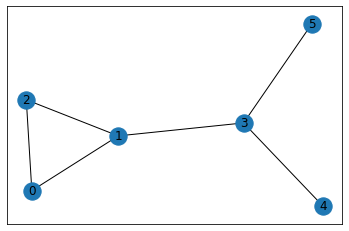Related Articles

# Visualize Graphs in Python

• Difficulty Level : Medium
• Last Updated : 03 Jul, 2020

Prerequisites: Graph Data Structure And Algorithms

A Graph is a non-linear data structure consisting of nodes and edges. The nodes are sometimes also referred to as vertices and the edges are lines or arcs that connect any two nodes in the graph.

In this tutorial we are going to visualize undirected Graphs in Python with the help of networkx library.

Installation:

To install this module type the below command in the terminal.

`pip install networkx`

Below is the implementation.

 `# First networkx library is imported ``# along with matplotlib``import` `networkx as nx``import` `matplotlib.pyplot as plt``  ` ` ` `# Defining a Class``class` `GraphVisualization:``  ` `    ``def` `__init__(``self``):``         ` `        ``# visual is a list which stores all ``        ``# the set of edges that constitutes a``        ``# graph``        ``self``.visual ``=` `[]``         ` `    ``# addEdge function inputs the vertices of an``    ``# edge and appends it to the visual list``    ``def` `addEdge(``self``, a, b):``        ``temp ``=` `[a, b]``        ``self``.visual.append(temp)``         ` `    ``# In visualize function G is an object of``    ``# class Graph given by networkx G.add_edges_from(visual)``    ``# creates a graph with a given list``    ``# nx.draw_networkx(G) - plots the graph``    ``# plt.show() - displays the graph``    ``def` `visualize(``self``):``        ``G ``=` `nx.Graph()``        ``G.add_edges_from(``self``.visual)``        ``nx.draw_networkx(G)``        ``plt.show()`` ` `# Driver code``G ``=` `GraphVisualization()``G.addEdge(``0``, ``2``)``G.addEdge(``1``, ``2``)``G.addEdge(``1``, ``3``)``G.addEdge(``5``, ``3``)``G.addEdge(``3``, ``4``)``G.addEdge(``1``, ``0``)``G.visualize()`

Output:Attention geek! Strengthen your foundations with the Python Programming Foundation Course and learn the basics.

To begin with, your interview preparations Enhance your Data Structures concepts with the Python DS Course. And to begin with your Machine Learning Journey, join the Machine Learning – Basic Level Course

My Personal Notes arrow_drop_up